# gkrecall

We brings you daily Current affairs, daily Current Affairs Quiz, weekly Current Affairs, weekly Current Affairs Quiz to enhance your preparation for upcoming exams. We also provide articles related to quant, reasoning, English, ssc etc .

# Arithmetic sum for RRB PO/CLERK And  RBI ASSISTANT MAINS

1. A train running at the speed of 72 km/hr can cross a pole and a bridge _________ meters long in _________ seconds and 24 seconds respectively. The values given in which of the following options will fill the blanks in the same order in which it is given to make the above statement true:

एक रेलगाड़ी 72 कि.मी./घंटा की गति से चल रही है और वह एक खंभे तथा एक .............. मीटर लंबे पुल को क्रमशः ..................... सेकण्ड और 24 सेकण्ड में पार करती है। निम्नलिखित विकल्प में दिए गए मान रिक्त स्थानों की क्रमशः पूर्ति करेंगे, कौन से विकल्प प्रश्न के अनुसार सही होंगे?

A) 320, 8

B) 280, 10

C) 250, 11

A. Only a

B. Only b and c

C. Only a and b

D. Only a and c

E. All a, b and c

2. A, B and C together can complete the work in 35 days. A and B together can complete the work in 60 days. If B is _________ % less efficient than C, then A alone can complete the work in __________ days. The values given in which of the following options will fill the blanks in the same order in which it is given to make the above statement true:

A, B और C मिलकर एक कार्य को 35 दिनों में पूरा कर सकते हैं। A और B मिलकर कार्य को 60 दिनों में पूरा कर सकते हैं। यदि B, C से .................. % कम दक्ष है, तब A कार्य को अकेले ................ दिनों में पूरा कर सकता है। निम्नलिखित विकल्प में दिए गए मान रिक्त स्थानों की क्रमशः पूर्ति करेंगे, कौन से विकल्प प्रश्न के अनुसार सही होंगे?

A) 20, 140

B) 30, 125

C) 40, 105

A. Only a

B. Only b

C. Only a and c

D. Only a and b

E. Only c

3. There are 60 ways of forming a group of (x + 2) men and 3 women out of a total of 6 men and 4 women. The values given in which of the following options will fill the blanks in the same order in which it is given to make the above statement true:

6 पुरुषों और 4 महिलाओं में से (x+2) पुरुष और 3 महिलाओं के एक समूह को चुनने की 60 विधियां हैं। निम्नलिखित विकल्प में दिए गए मान रिक्त स्थानों की क्रमशः पूर्ति करेंगे, कौन से विकल्प प्रश्न के अनुसार सही होंगे?

A) 0

B) 1

C) 2

D) 3

A. Only a

B. Only a and b

C. Only a and c

D. All a, b, c and d

E. None of a, b, c and d

4. A number series is given below, with some extra information. Answer the questions based on number series accordingly.

नीचे एक संख्या श्रृंखला कुछ अतिरिक्त जानकारी के साथ दी गयी है। उसके अनुसार संख्या श्रृंखला पर आधारित प्रश्नों के उत्तर दीजिए

127 254 508 1016 2032

x, _, _, __, ...... 10 terms.

Find the sum of the series, if x is the only positive root of equation: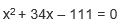यदि समीकरण:का केवल धनात्मक मूल है, तो श्रृंखला का योग ज्ञात कीजिए।

A. 3069

B. 2516

C. 4095

D. 5111

E. 1178

5. In the case of A, there is a mixture of milk and water (X + 24) liters in the ratio of 7: 8, whereas in B, the ratio of milk and water (X + 54) liters in the ratio of 3: 2 is B. If a mixture of 7 1/2% from the eligible A and 40% of the utensil mixture B is extracted and added to the eligible C Then the remaining mixture in the character B is 15 liters higher than the eligible A. Find the amount of milk in the eligible C?

पात्र में A में 7: 8 के अनुपात में दूध और पानी का (X + 24)लीटर मिश्रण है, जबकि पात्र B में 3 :2 के अनुपात में दूध और पानी का (X + 54) लीटर है। यदि पात्र A से 7 1/2%मिश्रण और बर्तन B से 40% मिश्रण निकाला जाता है और पात्र C में मिलाया जाता है। तो पात्र B में शेष मिश्रण पात्र A की तुलना में 15 लीटर अधिक है। पात्र C में दूध की मात्रा ज्ञात कीजिये?

A. 61 lit

B. 55 lit

C. 57 lit

D. 61 lit

E. None of these

Caselet

Directions (6 – 10): Read the following information carefully and answer the given questions.

In one of the college there are 1300 employees. The college has five departments – CSE, EEE, ECE, Civil and Mech. Out of the total number of female employees in the college, 30% work in CSE department, 22% work in ECE department, 18% work in EEE department, 12% work in Mech department and remaining 90 female employees work in Civil department. Out of the total number of male employees in the college, 18% work in CSE department, 20% work in ECE department, 30% work in Mech department, 22% work in EEE department and the remaining employees work in Civil department.

1) The total number of male employees working in the civil and EEE department together is approximately what percent of the total number of employees (both male and female) working in CSE and ECE department together?

a) 45%

b) 43%

c) 47%

d) 42%

e) 49%

2) If the male employees in ECE department is increase by 20%, the male employees in the Civil department increase by 10%, 25% of male employee increased by Mech department and 16 male employees Joins CSE department and the number of male employees in the EEE department is same, what is the percentage increase in the number of male employees in the college?

a) 12.5%

b) 14.5%

c) 16.5%

d) 10.5%

e) None of these

3) If 50 female employees from CSE department are transferred to the Mech department and 20 male employees from Mech department are transferred to the CSE department, what is the ratio of the number of female employee to the number of male employees in the Mech department after the transfer the employees?

a) 1: 2

b) 2: 5

c) 3: 2

d) 5: 3

e) None of these

4) What is the average number of employees (both male and female) who work in CSE, Mech and ECE department together?

a) 268

b) 258

c) 288

d) 298

e) None of these

5) What is the difference between the number of employees (both male and female) working in EEE department and the number of employees (both male and female) working in Civil department?

a) 76

b) 66

c) 86

d) 96

e) None of these

SOLUTIONS -

1. Speed of train = 72 km/hr = 20 m/s

For option a:

Length of train = 20 x 8 = 160

So, the length of the bridge = 20 x 24 – 160 = 320

480 – 160 = 320

320 = 320

So, option a can be the answer

For option b:

Length of train = 20 x 10 = 200

So, the length of the bridge = 20 x 24 – 200 = 280

480 – 200 = 280

280 = 280

So, option b can be the answer

For option c:

Length of train = 20 x 11 = 220

So, the length of the bridge = 20 x 24 – 220 = 250

480 – 220 = 250

260 not equal to 250

2. Let the total work = 420 units (LCM of 35 and 60)

Amount of work done by A, B and C together in one day =420/35=12units

Amount of work done by A and B together in one day =420/60=7units

Amount of work done by C alone in one day = 12 – 7 = 5 units

For option a:

Amount of work done by B alone in one day = 0.80 x 5 = 4 units

Amount of work done by A alone in one day = 7 – 4 = 3 units

Time taken by A alone to complete the work =420/3=140 days

So, option a can be the answer

For option b:

Amount of work done by B alone in one day = 0.70 x 5 = 3.5 units

Amount of work done by A alone in one day = 7 – 3.5 = 3.5 units

Time taken by A alone to complete the work =420/3.5=120 days

So, option b can’t be the answer

For option c:

Amount of work done by B alone in one day = 0.60 x 5 = 3 units

Amount of work done by A alone in one day = 7 – 3 = 4 units

Time taken by A alone to complete the work =420/4=105 days

So, option c can be the answer

3.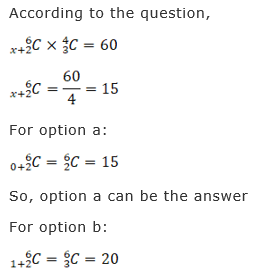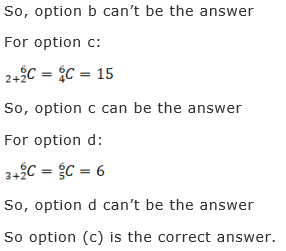So option (c) is the correct answer.

4.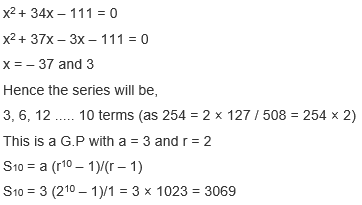5.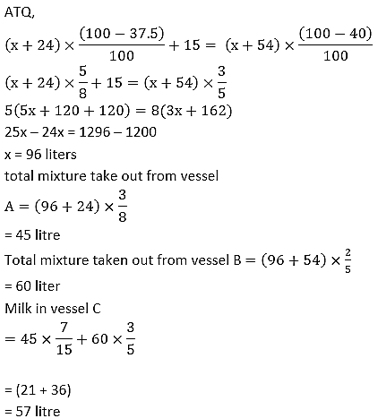Directions (6 – 10):

18% of female employees work in Civil department = 90

Total number of female employees in the college = 500

Number of female employees in CSE department = 500 * 30/100 = 150

Number of female employees in ECE department = 500 * 22/100 = 110

Number of female employees in EEE department = 500 * 18/100 = 90

Number of female employees in Mech department = 500 * 12/100 = 60

Total number of male employees in the college = 1300 – 500 = 800

Number of male employees in CSE department = 800 * 18/100 = 144

Number of male employees in EEE department = 800 * 22/100 = 176

Number of male employees in ECE department = 800 * 20/100 = 160

Number of male employees in Mech department = 800 * 30/100 = 240

Number of male employees in Civil department = 800 * 10/100 = 80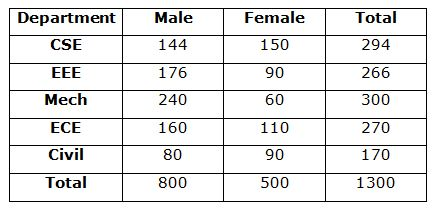Number of male employee in Civil department = 80

Number of male employee in EEE department = 176

Total male of Civil and EEE = 256

Total number of employees in CSE department = 294

Total number of employees in ECE department = 270

Total employees of ECE and CSE = 564

Required percentage = 256 * 100/564 = 45%

Number of male employees in ECE department = 160 * 120/100 = 192

Number of male employees in Civil department = 80 * 110/100 = 88

Number of male employees in Mech department = 240 * 125/100 = 300

Number of male employees in CSE department = 144 + 16 = 160

Number of male employees in EEE department = 176

New total number of male employees in the college = 916

Required percentage = (916 – 800) * 100/800 = 14.5%

After transferred the number of female employees in Mech department

= 60 + 50 = 110

After transferred number of male employees in Mech department

= 240 – 20 = 220

Required ratio = 110: 220 = 1: 2